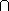# ANALYSIS II Metric Spaces: Open and Closed Sets

Defn   If> 0, then an open-neighborhood of x is defined to be the set B(x) := {y in X | d(x,y) <}. This set is also referred to as the open ball of radiusand center x. The set {y in X | d(x,y)}is called the closed ball, while the set {y in X | d(x,y) =}is called a sphere.

Defn  A subset O of  X is called open if, for each x in O, there is an-neighborhood of x which is contained in O.

Proposition  Each open-neighborhood in a metric space is an open set.

Theorem The following holds true for the open subsets of a metric space (X,d):

1. Both X and the empty set are open.
2. Arbitrary unions of open sets are open.
3. Finite intersections of open sets are open. (Homework due Wednesday)

Proposition  Suppose Y is a subset of X, and dY is the restriction of d to Y, then

1. (Y,dY) is a metric space and open subsets of Y are just the intersections with Y of open subsets of X.
2. if Y is open in X, a set is open in Y if and only if it is open in X.
3.  in general, open subsets relative to Y may fail to be open relative to X.

Examples:  Arbitrary intersectons of open sets need not be open:

1. If  On := (-1/n, 1/n), thenn On = {0}.
2. If  On := (-1/n, 1 +1/n), thenn On = [0,1).

Defn  Suppose X is a set and T is a collection of subsets of X with the properties

1. both X and the empty set belong to T,
2. T is closed under arbitrary unions,
3. T is closed under finite intersections,

then (X, T ) is called a topological space and T is called a topology for X. Moreover, each O in T is called a neighborhood for each of their points.

Defn  A subset C of a metric space X is called closed if its complement is open in X.

Examples:  Each of the following is an example of a closed set:

1. Each closed-nhbd is a closed subset of X.
2. The set {x in R | xd } is a closed subset of C.
3. Each singleton set {x} is a closed subset of X.
4. The Cantor set is a closed subset of R.

To construct this set, start with the closed interval [0,1] and recursively remove the open middle-third of each of the remaining closed intervals ...
At the n-th stage, we have 2n closed intervals each of length (1/3)n :

Stage 0:                      [0,1]
Stage 1:        [0,1/3]                [2/3,1]
Stage 2:  [0,1/9]  [2/9,3/9] [6/9,7/9] [8/9,1]
.
.
.

This finite union of closed intervals is closed. The Cantor set is the intersection of this (decreasing or nested) sequence of sets and so is also closed. Later, we will see that the Cantor set has many other interesting properties.

Homework #4 (Due Monday 01/26)

1. Suppose (X,T) is a topological space. Prove each of the following:
1. Both X and empty set are closed sets.
2. Arbitrary intersections of closed sets are closed.
3. Finite unions of closed sets are closed.
2. Show that {0 , 1 , 1/2 , 1/3 , ... , 1/n , ...} is a closed set in R and in C.

Robert Sharpley Jan 19 1998